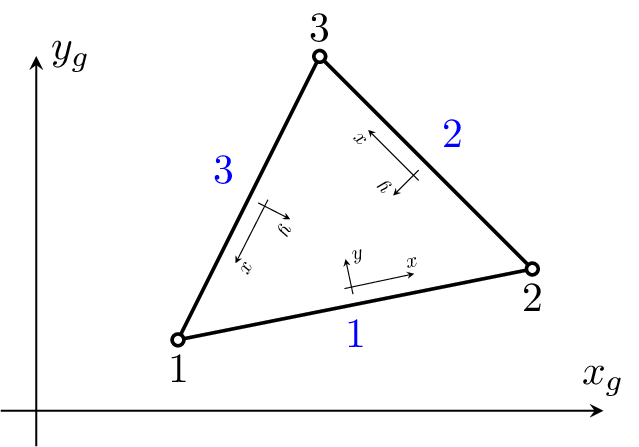#### 4.4 3D SUPG/PSGP Elements

##### 4.4.1 Tet1_3D_SUPG element

Represents 3D linear pyramid element for transient incompressible flow analysis using SUPG/PSPG stabilization with equal order approximation of velocity and pressure fields. Each node has 3 degrees of freedoms (two components of velocity and pressure). The element features are summarized in Table 64.Figure 29: Tet1_3D_SUPG element.

 Keyword TET1SUPG Description linear equal order approximation axisymmetric element Specific parameters [vof #(rn)][pvof #(rn)] Unknowns Three velocity components (V_u, V_v, and V_w) and pressure (P_f) are required in each node. Approximation Linear approximation of velocity and pressure fields. Integration exact Loads Constant boundary tractions are supported. Body loads representing the self-weight load are supported. Multi-fluid analysis The element has support for solving problems with two immiscible fluids in a fixed spatial domain. In the present implementation, a LevelSet tracking algorithm is used to track the position of interface. The material model should be of type Keyword: twofluidmat, that supports modelling of two immiscible fluids. Status

Table 64: TET1SUPG element summary